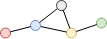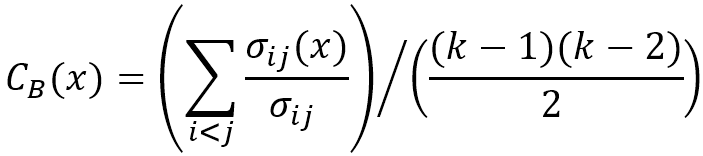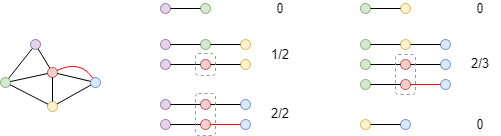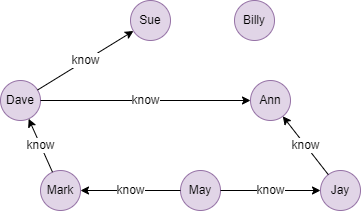# Change Nickname

Current Nickname:

Certifications

Certificate Issued at Valid until Serial No. File
Serial No. Valid until

Not having one? Apply now! >>>

Invoice

ProductName CreateTime ID Price File
ProductName CreateTime ID Price File
v4.0
Search
中文EN
v4.0

# Betweenness Centrality

## Overview

Betweenness centrality is used to measure the probability that a node appears in the shortest paths between any other two nodes. Proposed by Linton C. Freeman in 1977, this concept can effectively calculate the nodes that play an important role as bridge and medium between multiple parts of the graph.

The range of values of betweenness centrality is [0,1]; the larger the value, the stronger the mediating effect.

Related materials are as below:

## Basic Concept

### Betweenness Probability

Betweenness in this algorithm refers to the nodes that a node passes through when it reaches another node through the shortest paths.In the graph above, the shortest path between the red and green nodes passes through the blue and yellow two nodes, the blue and yellow nodes are both their in-between nodes.

Betweenness probability is the probability that a node lies on all the shortest paths of a node pair.In the graph above, there are three 3-step shortest paths between the red and green nodes, two of which have the yellow node, so the betweenness probability of the yellow node for the red-green node pair is `2 / 3 = 0.6667`.

For the case of two nodes from different connected components in an unconnected graph, any other node in the graph has a zero betweenness probability for this node pair.

### Betweenness Centrality

All node pairs except the target node in the graph have to be listed out before calculating betweenness centrality, then to calculate the betweenness probability of the target node for each node pair, and to average those probabilities:where `x` is the node to be calculated, `i` and `j` are any two distinct nodes (`x` is excluded) in the graph, `i` and `j` are paired without repeating, `σ` is the number of all the shortest paths between `i` and `j`, `σ(x)` is the number of the shortest paths that involve node `x`, `σ(x)`/`σ` is the betweenness probability of `x` for node pair `i` and `j` (which is zero if `i` and `j` are not connected), `k` is the number of nodes contained in the graph, that is, `(k-1)(k-2)/2` is the number of `i` and `j` node pairs.Calculate the betweenness centrality of the red node in the graph above. Calculate the betweenness probabilities of the red node for each node pair according to the shortest paths, and average them to obtain betweenness centrality as `(1/2 + 1 + 2/3) / 6 = 0.3611`.

Betweenness centrality algorithm consumes considerable computing resources as it calculates the number of all the shortest paths that involve a node in the whole graph. You may perform sampling betweenness centrality calculation on GraphSet with more than 10,000 nodes, the suggested number of the samples is the logarithm based on 10 `log(number of nodes)`; user can configure whether to sample or not.

## Special Case

### Lonely Node, Disconnected Graph

Lonely node is not connected with any other node, so its betweenness centrality is 0. Lonely node is also paired with other nodes and affects the number of node pairs in the graph.

For disconnected graph, two nodes from different connected components always give a zero betweenness probability for any other node in the graph.

### Self-loop Edge

It is the shortest distance between nodes that betweenness centrality calculates, self-loop edge does not constitute the shortest path, thus it does not participate in the calculation.

### Directed Edge

For directed edges, betweenness centrality algorithm ignores the direction of edges but calculates them as undirected edges.

## Results and Statistics

Take the 7-people small social network below as an example, run the Betweenness Centrality algorithm against all nodes:Algorithm results: Calculate betweenness centrality for each node, return `_id`, `centrality` or `_uuid`, `centrality` according to the execution method

_uuid _id centrality
1 Sue 0.0000000
2 Dave 0.33333334
3 Ann 0.13333334
4 Mark 0.13333334
5 May 0.066666670
6 Jay 0.066666670
7 Billy 0.0000000

Algorithm statistics: N/A

## Command and Configuration

• Command: `algo(betweenness_centrality)`
• Configurations for the parameter `params()` :
Name
Type
Default
Specification
Description
sample_size int -1 -1, -2 or (0,Number of nodes] Number of nodes to be sampled, -1 means to sample` log(<number of nodes in the whole graph)` nodes, no sampling is applied and use all nodes to calculate precisely if sets to -2 or not set
limit int -1 >=-1 Number of results to return; return all results if sets to -1 or not set
order string / ASC/DESC, case insensitive To sort the returned results; no sorting is applied if not set

Example: Precisely calculate betweenness centrality of all nodes without sampling, and return 6 results

``````algo(betweenness_centrality).params({
limit: 6,
sample_size: -2
}) as bc
return bc
``````

## Algorithm Execution

#### 1. File Writeback

Configuration Data in Each Row
filename `_id`,`centrality`

Example: Calculate betweenness centrality of all nodes, write the algorithm results back to file named cen

``````algo(betweenness_centrality).params().write({
file:{
filename: "cen"
}
})
``````

#### 2. Property Writeback

Configuration Writeback Content Type Data Type
property `centrality` Node property `float`

Example: Calculate betweenness centrality of all nodes, write the centrality back to node property named bc

``````algo(betweenness_centrality).params().write({
db:{
property: "bc"
}
})
``````

#### 3. Statistics Writeback

This algorithm has no statistics.

### Direct Return

Alias Ordinal Type
Description
Column Name
0 []perNode Node and its betweenness centrality `_uuid`, `centrality`

Example: Calculate betweenness centrality of all nodes, define algorithm results as alias named results, nd return the 3 results with the highest centrality

``````algo(betweenness_centrality).params({
order: "desc",
limit: 3
}) as results
return results
``````

### Streaming Return

Alias Ordinal Type
Description
Column Name
0 []perNode Node and its betweenness centrality `_uuid`, `centrality`

Example: Calculate the number of nodes whose betweenness centrality is 0

``````algo(betweenness_centrality).params().stream() as results
where results.centrality == 0
return count(results)
``````

### Real-time Statistics

This algorithm has no statistics.